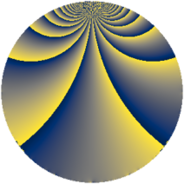# Properties

 Label 135.2.pLevel $135$ Weight $2$ Character orbit 135.p Rep. character $\chi_{135}(4,\cdot)$ Character field $\Q(\zeta_{18})$ Dimension $96$ Newform subspaces $1$ Sturm bound $36$ Trace bound $0$

# Related objects

## Defining parameters

 Level: $$N$$ $$=$$ $$135 = 3^{3} \cdot 5$$ Weight: $$k$$ $$=$$ $$2$$ Character orbit: $$[\chi]$$ $$=$$ 135.p (of order $$18$$ and degree $$6$$) Character conductor: $$\operatorname{cond}(\chi)$$ $$=$$ $$135$$ Character field: $$\Q(\zeta_{18})$$ Newform subspaces: $$1$$ Sturm bound: $$36$$ Trace bound: $$0$$

## Dimensions

The following table gives the dimensions of various subspaces of $$M_{2}(135, [\chi])$$.

Total New Old
Modular forms 120 120 0
Cusp forms 96 96 0
Eisenstein series 24 24 0

## Trace form

 $$96 q - 12 q^{4} - 9 q^{5} - 6 q^{6} - 18 q^{9} + O(q^{10})$$ $$96 q - 12 q^{4} - 9 q^{5} - 6 q^{6} - 18 q^{9} - 3 q^{10} - 6 q^{11} - 18 q^{14} - 21 q^{15} - 24 q^{16} - 6 q^{19} - 57 q^{20} + 24 q^{21} - 30 q^{24} + 3 q^{25} + 48 q^{26} - 30 q^{29} - 51 q^{30} - 30 q^{31} - 24 q^{34} - 12 q^{35} + 54 q^{36} - 6 q^{39} - 9 q^{40} - 12 q^{41} + 78 q^{44} + 45 q^{45} - 6 q^{46} - 30 q^{49} + 84 q^{50} - 90 q^{51} + 108 q^{54} - 12 q^{55} - 96 q^{56} + 66 q^{59} + 84 q^{60} + 6 q^{61} + 45 q^{65} - 150 q^{66} + 24 q^{69} - 33 q^{70} - 90 q^{71} + 66 q^{74} + 39 q^{75} + 12 q^{76} + 24 q^{79} + 30 q^{80} - 54 q^{81} + 198 q^{84} - 21 q^{85} + 18 q^{86} + 96 q^{89} + 90 q^{90} - 6 q^{91} + 24 q^{94} + 87 q^{95} + 42 q^{96} + 36 q^{99} + O(q^{100})$$

## Decomposition of $$S_{2}^{\mathrm{new}}(135, [\chi])$$ into newform subspaces

Label Dim $A$ Field CM Traces $q$-expansion
$a_{2}$ $a_{3}$ $a_{5}$ $a_{7}$
135.2.p.a $96$ $1.078$ None $$0$$ $$0$$ $$-9$$ $$0$$